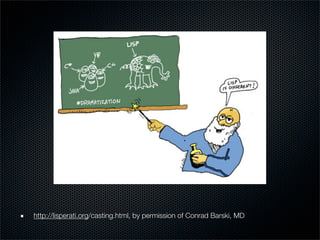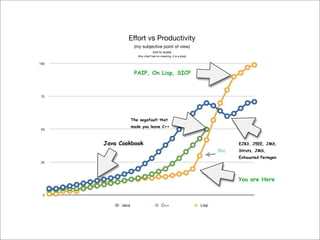Successfully reported this slideshow.

# Introduction To Lisp

21

Share×
1 of 52
1 of 52

# Introduction To Lisp

21

Share

Introduction to Lisp. A survey of lisp's history, current incarnations and advanced features such as list comprehensions, macros and domain-specific-language [DSL] support.

Introduction to Lisp. A survey of lisp's history, current incarnations and advanced features such as list comprehensions, macros and domain-specific-language [DSL] support.

## More Related Content

### Related Books

Free with a 14 day trial from Scribd

See all

### Related Audiobooks

Free with a 14 day trial from Scribd

See all

### Introduction To Lisp

1. 1. Why Won’t Lisp Just Go Away? Inroducing Lisp 50 Years Young (2008)
2. 2. http://lisperati.org/casting.html, by permission of Conrad Barski, MD
3. 3. Effort vs Productivity (my subjective point of view) (not to scale) (this chart has no meaning, it is a joke) 100 PAIP, On Lisp, SICP 75 The segafault that made you leave C++ 50 Java Cookbook EJB3, J5EE, JMX, Me Struts, JMS, Exhausted Permgen 25 You are Here 0 Java C++ Lisp
4. 4. Overview What is Lisp? How to get started Some Basics Some Common Recipes Wet your appetite for more Not so basic things Where to go next
5. 5. What is Lisp? A Programming Language Brought to you by John McCarthy circa 1958 Resistance is Futile... Garbage Collection, Closures, Functional, Object Oriented, AOP, DSLs, Conditions and Restarts, Continuations, what else ya got?
6. 6. Welcome to the Family Common Lisp (Lisp 2) LispWorks, Alegro OpenMCL, SBCL, Clisp, ABCL and others Scheme (Lisp 1) by Sussman and Steele PLT, Chicken, Bigloo, Clojure, SISC, JScheme, and others Less Common Dylan, Qi, Termite, ECL, Arc, and others...
7. 7. Quick Start LispBox http://gigamonkeys.com/lispbox/ Windows, OS X, Linux Ready Lisp http://www.newartisans.com/software/readylisp.html OS X
8. 8. Roll your own Emacs SLIME The Superior Lisp Interaction Mode for Emacs Common Lisp SBCL, CLISP paraedit-el
9. 9. Basics Parentheses, lots and lots of parentheses Everything is an Expression Preﬁx Notation Lists, ‘cons’, pair Singly Linked List
10. 10. Basics: Cons Cells, Lists car (), nil, the empty list, false cdr 1 (list 1) (cons 1 nil) 1 2 (cons 1 2) 1 2 3 (list 1 2 3) (cons 1 (cons 2 (cons 3 nil)))
11. 11. Basics: Expressions (if test-p consequent otherwise) (+ 1 2 3 4 5) => 15 (let ((x 3) (y 2)) (* x y)) => 6 (expt 2 42) => 4398046511104
12. 12. Basics: Functions (defun test (n) (cond ((= n 0) (+ 1 n)) (t (- n 1)))) (funcall #'test 3) (apply #’test ‘(3)) (mapcar #'test '(-2 -1 0 1 2)) => (-3 -2 1 0 1)
13. 13. Basics: Assignment (setf x '(a b c)) => (A B C) (setf (car x) 1) => (1 B C) (setf (second x) 2) => (1 2 C)
14. 14. Numeric Tower Ints and Floats, but also... Ratios Built in Bignum Support Built in Complex Numbers
15. 15. Numeric Tower 1/2 (/ 1 3) => 1/3 (* 33333 (/ 1 33333)) => 1 (expt 2 100) => 1267650600228229401496703205376 (* #c(0 1) #c(0 1)) => -1
16. 16. Familiar Data Structures Vectors (arrays) Hash Tables (map) Structures (records) Objects - CLOS Sequence Types (and streams)
17. 17. Library System: ASDF ASDF - another system deﬁnition facility Like Ruby’s gem or Perl’s CPAN (but inscrutable) (require :asdf-install) (asdf-install:install :cl-ppcre) Repository: cliki.net
18. 18. Example: Fetch the Web (require :drakma) (use-package :drakma) (defvar content nil) (setf content (http-request quot;http://google.com/quot;)) (format t quot;len:~a~&quot; (length content)) (format t quot;~a~&quot; (subseq content 0 100))
19. 19. Example: Serve the Web (require :hunchentoot) (use-package :hunchentoot) (setq *dispatch-table* (list (create-prefix-dispatcher quot;/indexquot; 'index-page))) (defun index-page () quot;<h1>Hunchentoot Demo</h1>.quot;) (defvar server (hunchentoot:start-server :port 4242))
20. 20. Example: Process Files (require :cl-ppcre) (with-open-file (inp quot;.../input.txtquot; :direction :input) (loop for line = (read-line inp nil nil) while line do (format t quot;~a~&quot; (cl-ppcre:regex-replace quot;lis+pquot; line quot;lispquot;))))
21. 21. Example: Unit Testing (define-test test-flatten (assert-false (flatten)) (assert-false (flatten '())) (assert-equal '(a) (flatten 'a)) (assert-equal '(a) (flatten '(a))) (assert-equal '(a b c d) (flatten '(a (b (c (d)))))))
22. 22. More Interesting Stuff Macros, Reader Macros, syntax-rules Restarts “To Err is Expected, To Recover, Divine” -- David B. Lamkins Functional Programming Pattern Matching (Uniﬁcation)
23. 23. What’s a Macro? A tool for Abstraction Code Generator Compiler defmacro deﬁne-macro syntax-rules
24. 24. Macro: random-if (defmacro random-if (prob consequent otherwise) `(if (< (random 1.0) ,prob)) ,consequent ,otherwise)) (random-if 1/2 (format t quot;went left~&quot;) (format t quot;went right~&quot;)
25. 25. Macro: aprog1 (defmacro aprog1 (it &rest body) `(let ((it ,it)) give the thing a name ,@body let them use it it)) return it (aprog1 (make-hash-table :test #’equal) (setf (gethash “a” it) 1) (setf (gethash “b” it) 2)) => #<HASH-TABLE :TEST EQUAL :COUNT 2 {BE49831}>
26. 26. Macro: rcut (defmacro rcut (&rest body)  (let* ((formals (list))         (new-body          (map-tree #'(lambda (elt)                        (if (symbol-equal '<> elt)                            (aprog1                             (gensym)                             (push it formals)                             it)                            elt))                    body)))    `#'(lambda ,(reverse formals)         ,@new-body))) (macroexpan-1 ‘(rcut (format “val:~a~&” <>))) => #'(LAMBDA (#:G2348) (FORMAT NIL quot;elt:~a~&quot; #:G2348))
27. 27. Restarts: Structured Recovery (defun file-size (file) (restart-case (if (not (probe-file file)) ‘throw’ (error 'file-not-found) (sb-posix:stat-size (sb-posix:stat file))) (file-not-found (new-file-size) :report quot;Specify missing size.quot; recovery :interactive read-new-value new-file-size))) (defun bytes-used (files) (loop for file in files summing (file-size file)))
28. 28. Restarts in action CL-USER> (format t quot;bytes:~a~&quot; (bytes-used '(quot;/etc/passwdquot; quot;/foo/barquot;))) Condition FILE-NOT-FOUND was signalled. [Condition of type FILE-NOT-FOUND] Restarts: 0: [FILE-NOT-FOUND] Specify missing size. 1: [ABORT] Return to SLIME's top level. 2: [TERMINATE-THREAD] Terminate this thread (#<THREAD quot;repl-threadquot; ...>) Backtrace: 0: (FILE-SIZE quot;/foo/barquot;) 1: (BYTES-USED (quot;/etc/passwdquot; quot;/foo/barquot;)) ...snip... Enter a new value: 12 bytes:2900
29. 29. More Interesting Stuff Functional Programming Functions are ﬁrst class - composable list comprehensions (cl-incf) pattern-matching (cl-uniﬁcation, pcond) DSLs, mini-languages
30. 30. First Class Functions (mapcar #'(lambda (x) (+ 2 x)) '(1 2 3 4)) => ‘(3 4 5 6) (defun curry (fn &rest args) #'(lambda (&rest rest) (apply fn (append args rest)))) (funcall (curry #'+ 2) 3) => 5 (mapcar (curry #'+ 2) '(1 2 3 4)) => ‘(3 4 5 6)
31. 31. First Class Functions (defun memoize (fn) (let ((cache (make-hash-table :test #'equal))) #’(lambda (&rest args) (let ((key (format nil quot;~aquot; args))) (if (gethash key cache) Return (gethash key cache) from cache (aprog1 (apply fn args) (setf (gethash key cache) it))))))) Add result to cache and return
32. 32. First Class Functions (defun myfn (a b c) (format t quot;called: a:~a b:~a c:~a~&quot; a b c) (+ a b c)) (let ((mfn (memoize #'myfn)) (args '(1 2 3)) (args2 '(4 5 6))) (format t quot;mfn ~a: ~a~&quot; args (apply mfn args)) (format t quot;mfn ~a: ~a~&quot; args (apply mfn args)) (format t quot;mfn ~a: ~a~&quot; args2 (apply mfn args2)) (format t quot;mfn ~a: ~a~&quot; args2 (apply mfn args2))) => called: a:1 b:2 c:3 not in cache mfn (1 2 3): 6 mfn (1 2 3): 6 in cache called: a:4 b:5 c:6 not in cache mfn (4 5 6): 15 mfn (4 5 6): 15 in cache
33. 33. List Comprehensions (defun lcmp-qsort (things) (cond ((null things) things) (t (destructuring-bind (pivot . tl) things (append (lcmp-qsort (assemble x (<- x tl) (< x pivot))) (list pivot) (lcmp-qsort (assemble x (<- x tl) (>= x pivot))))))))
34. 34. List Comprehensions (defun lcmp-qsort (things) (cond ((null things) things) (t (destructuring-bind (pivot . tl) things (append (lcmp-qsort (assemble x (<- x tl) (< x pivot))) (list pivot) (lcmp-qsort (assemble x (<- x tl) (>= x pivot))))))))
35. 35. List Comprehensions (defun lcmp-qsort (things) (cond ((null things) things) (t (destructuring-bind (pivot . tl) things (append (lcmp-qsort (assemble x (<- x tl) (< x pivot))) (list pivot) (lcmp-qsort (assemble x (<- x tl) (>= x pivot))))))))
36. 36. List Comprehensions (defun lcmp-qsort (things) (cond ((null things) things) (t (destructuring-bind (pivot . tl) things (append (lcmp-qsort (assemble x (<- x tl) (< x pivot))) (list pivot) (lcmp-qsort (assemble x (<- x tl) (>= x pivot))))))))
37. 37. List Comprehensions (defun lcmp-qsort (things) (cond ((null things) things) (t (destructuring-bind (pivot . tl) things (append (lcmp-qsort (assemble x (<- x tl) (< x pivot))) (list pivot) (lcmp-qsort (assemble x (<- x tl) (>= x pivot))))))))
38. 38. List Comprehensions (defun lcmp-qsort (things) (cond ((null things) things) (t (destructuring-bind (pivot . tl) things (append (lcmp-qsort (assemble x (<- x tl) (< x pivot))) (list pivot) (lcmp-qsort (assemble x (<- x tl) (>= x pivot))))))))
39. 39. List Comprehensions (defun all-permutations (things) (cond ((= 1 (length things)) (list things)) (t (assemble (cons Head Tail) (<- Head things) (<- Tail (all-permutations (remove Head things)))))))
40. 40. List Comprehensions (defun all-permutations (things) (cond ((= 1 (length things)) (list things)) (t (assemble (cons Head Tail) (<- Head things) (<- Tail (all-permutations (remove Head things)))))))
41. 41. List Comprehensions (defun all-permutations (things) (cond ((= 1 (length things)) (list things)) (t (assemble (cons Head Tail) (<- Head things) (<- Tail (all-permutations (remove Head things)))))))
42. 42. List Comprehensions (defun all-permutations (things) (cond ((= 1 (length things)) (list things)) (t (assemble (cons Head Tail) (<- Head things) (<- Tail (all-permutations (remove Head things)))))))
43. 43. List Comprehensions (defun all-permutations (things) (cond ((= 1 (length things)) (list things)) (t (assemble (cons Head Tail) (<- Head things) (<- Tail (all-permutations (remove Head things)))))))
44. 44. List Comprehensions (defun all-permutations (things) (cond ((= 1 (length things)) (list things)) (t (assemble (cons Head Tail) (<- Head things) (<- Tail (all-permutations (remove Head things)))))))
45. 45. Pattern Matching: pcond (defun ptest (names) (pcond:pcond ((and (:pat ((?first ?last) . ?rest) names) (:re quot;(.+)oquot; ?first (before-o))) (format t quot;before-o: ~a~&quot; before-o) (ptest ?rest)))) (ptest '((quot;George quot; quot;Washingtonquot;) (quot;Johnquot; quot;Adamsquot;))) => before-o: Ge => before-o: J
46. 46. DSLs, mini-languages (define-syntax aprog1 (syntax-rules (it) ((_ expression . body) (let ((it expression)) body it)))) (macroexpand '(aprog1 (list) (set! it (cons ‘a it)))) => (let ((it (list))) (set! it (cons 'a it)) it)
47. 47. DSLs, mini-languages (define m (automation init (init : (c -> more)) (more : (a -> more) (d -> more) (r -> end)) (end :)))
48. 48. DSLs, mini-languages (define-syntax automation (syntax-rules (: ->) ((_ init-state (state : (label -> target) ...) ...) (letrec ((state (lambda (stream) (cond ((empty? stream) #t) (else (case (first stream) ((label) (target (rest stream))) ... (else #f)))))) ...) init-state))))
49. 49. DSLs, mini-languages (letrec ((init (lambda (GenTemp%2) (cond ((empty? GenTemp%2) #t) (else (case (first GenTemp%2) ((c) (more (rest GenTemp%2))) (else #f)))))) (more (lambda (GenTemp%2) (cond ((empty? GenTemp%2) #t) (else (case (first GenTemp%2) ((a) (more (rest GenTemp%2))) ((d) (more (rest GenTemp%2))) ((r) (end (rest GenTemp%2))) (else #f)))))) (end (lambda (GenTemp%2) (cond ((empty? GenTemp%2) #t) (else (case (first GenTemp%2) (else #f))))))) init)
50. 50. That’s the overview http://lisperati.org/casting.html, by permission of Conrad Barski, MD
51. 51. Now where am I supposed to go? On-Line Resources Common Lisp Hyper Spec On Lisp (Paul Graham) Practical Common Lisp (Peter Siebel) Cliki.net common-lisp.net JRMs Syntax Rules Primer Casting Spells with Lisp: http://lisperati.org/casting.html http://del.icio.us/mortis/lisp
52. 52. Now where am I supposed to go? Books PAIP (Peter Norvig) Practical Common Lisp (Peter Seibel) SICP (Ableson and Sussman) Common Lisp the Language (Guy Steele) ANSI Common Lisp (Paul Graham)